﻿ Appendix 6A: Bond Immunization Theoremoffice (412) 9679367
fax (412) 967-5958
toll-free 1 (800) 214-3480

Appendix 6A:

Bond Immunization Theorem: A Closer Look

The bond immunization theorem (first derived by Samuelson) states that interest rate risk can be hedged by matching the duration of a bond with the desired holding period.

The proof of this theorem exploits the fact that bond prices move inversely with interest rate risk, but the reinvestment of coupon payments can be made at higher rates. First, recall the example provided in Topic 6.4, reproduced in this appendix for your convenience:

Alternative A:

At the end of ten years, the cash flow is \$109. There is no other cash flow.

Alternative B:

There is a cash flow of \$22 at the end of Year 2 and a cash flow of \$135 at the end of Year 18. There are no other cash flows.

Alternative A has a duration of 10. If the interest rate is 12%, then Alternative B also has a duration of 10. Note that even though we know the duration of each component of Alternative B (2 and 18), we cannot determine the duration of the two cash flows together without reference to the interest rate. At "today’s" interest rate of 12%, both these cash flows have the same present value. The present value as a function of the interest rate is given in Table 6A.1.

Table 6A.1 Present Values of Alternatives A and B

 Interest Rate PV(A) PV(B) Duration of B PV(B) -PV(A) 0.05 66.92 76.05 13.80 9.13 0.06 60.86 66.88 13.32 6.02 0.07 55.41 59.16 12.80 3.75 0.08 50.49 52.65 12.27 2.16 0.09 46.04 47.14 11.71 1.10 0.10 42.02 42.46 11.15 0.44 0.11 38.39 38.49 10.58 0.10 0.12 35.09 35.09 10.00 0.00 0.13 32.11 32.19 9.44 0.08 0.14 29.40 29.69 8.88 0.29 0.15 26.94 27.54 8.34 0.60 0.16 24.71 25.68 7.81 0.97 0.17 22.68 24.07 7.32 1.39 0.18 20.83 22.66 6.84 1.83

Note that the present values are equal at 12%, where the durations are also equal. Otherwise, at other interest rates, Alternative B is always more valuable than Alternative A.

We know the two alternatives have the same value and the same duration at r = 12%. This means that the two curves must touch at the point (r,P), where r = 12% and P = \$35.09. We also know that the price is inversely related to the yield, so both the curves are negatively sloped. We now show that the second derivative of the first is smaller than the second derivative of the second if the bonds have the same duration. This implies that the curves must be tangent at point (r,P), and the second must lie above the first, exactly as shown.

Recall that,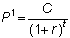The results for the second derivative follow from Jensen’s inequality, as follows: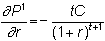and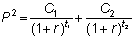so that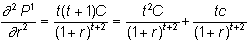and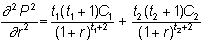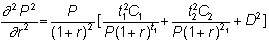To see that the first term exceeds the second, recall from the definition of duration that if we let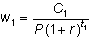andthen w1 + w2 = 1, and because the durations of the two bonds are equal, we also get

t = t1w1 + t2w2

Therefore, if we can show that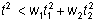we will have shown that the second derivative of the first bond is smaller than that of the second. This follows from the fact that t2 is a convex function and from Jensen's inequality. The inequality says that if f(x) is a strictly convex function of x, then f(ax1 + (1 - a)f(x2. If we let a= w1, 1 - a = w2, and x = t, the result follows.